# Question 25 Given the equation 7x2 + 4y2 - 28 = 0. Determine it vertices, as...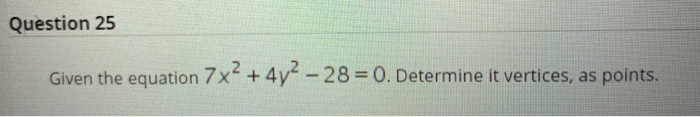Question 25 Given the equation 7x2 + 4y2 - 28 = 0. Determine it vertices, as points.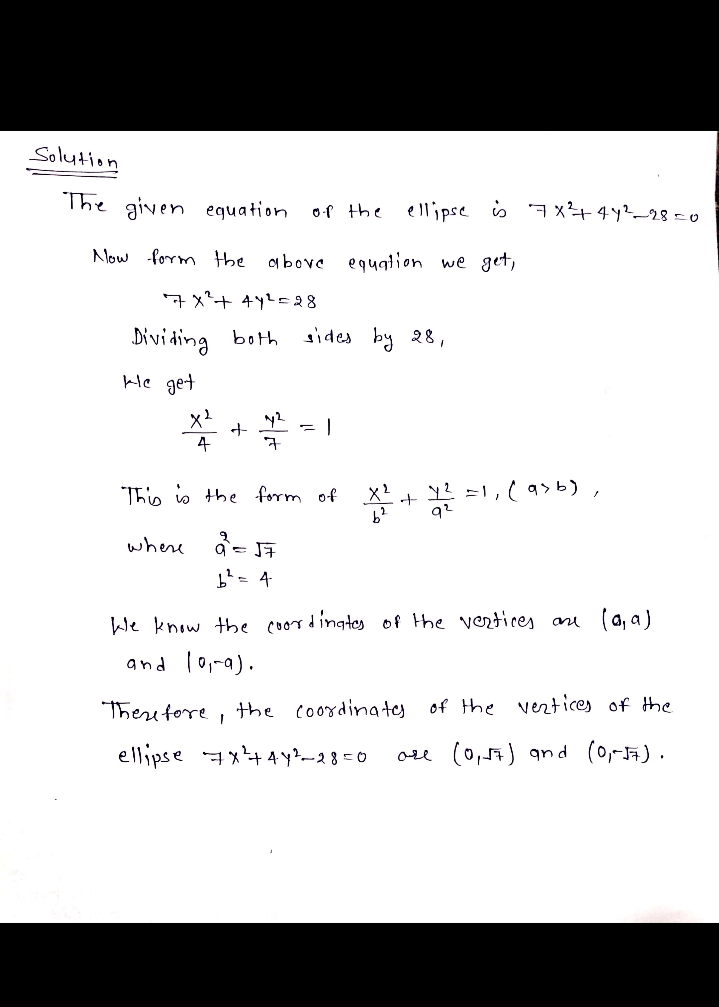##### Add Answer of: Question 25 Given the equation 7x2 + 4y2 - 28 = 0. Determine it vertices, as...
Similar Homework Help Questions
• ### Question 24 Given that f(x) = – In(x-3)+ 1. Determine its domain, in interval notation. Question...Question 24 Given that f(x) = – In(x-3)+ 1. Determine its domain, in interval notation. Question 25 Given the equation 7x2 + 4y? – 28 = 0. Determine it vertices, as points.

• ### Find an equation for the hyperbola that satisfies the given conditions. Foci: (0, +6), vertices: (0,...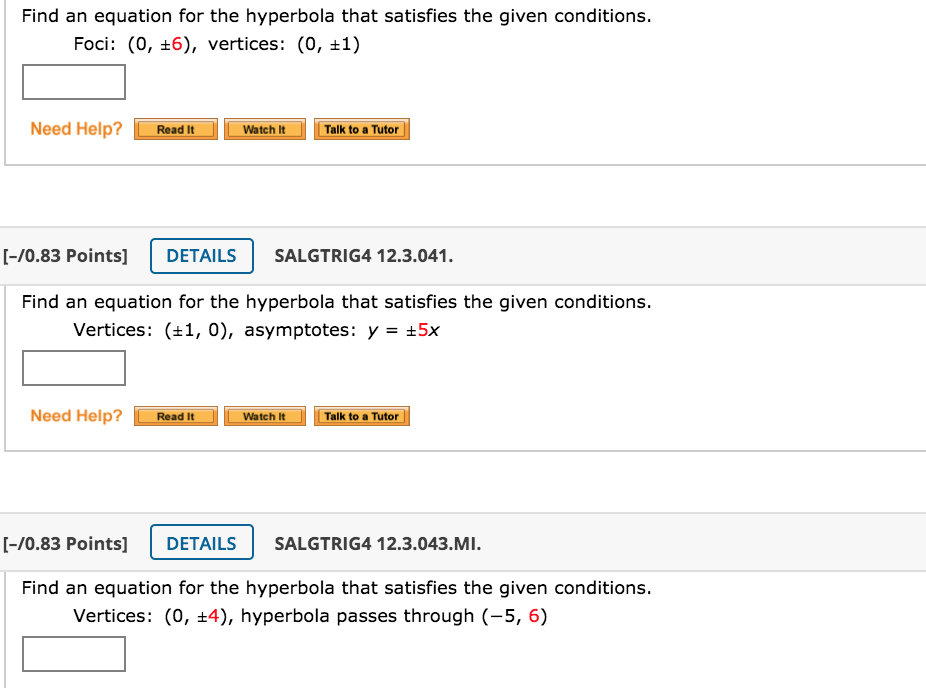Find an equation for the hyperbola that satisfies the given conditions. Foci: (0, +6), vertices: (0, +1) Need Help? Read It Watch It Talk to a Tutor [-70.83 Points] DETAILS SALGTRIG4 12.3.041. Find an equation for the hyperbola that satisfies the given conditions. Vertices: (+1,0), asymptotes: y = 5x Need Help? Read It Watch It Talk to a Tutor [-70.83 Points] DETAILS SALGTRIG4 12.3.043.MI. Find an equation for the hyperbola that satisfies the given conditions. Vertices: (0, +4), hyperbola passes...

• ### Determine graphically the vertices of the triangle, the equation of whose sides are given as y = x; y = 0; 2x + 3y = 10

Determine graphically the vertices of the triangle, the equation of whose sides are given as y = x; y = 0; 2x + 3y = 10. Interpret the result.

• ### Question 5 (25 points) : Use Lagrange multipliers to find all the critical points of the function f (x, y, z)-22on the surface 2 -4y2 722. You do not need to determine whether they are maxima, minima...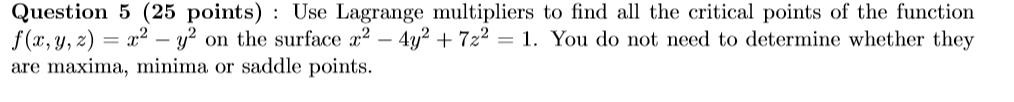Question 5 (25 points) : Use Lagrange multipliers to find all the critical points of the function f (x, y, z)-22on the surface 2 -4y2 722. You do not need to determine whether they are maxima, minima or saddle points. Question 5 (25 points) : Use Lagrange multipliers to find all the critical points of the function f (x, y, z)-22on the surface 2 -4y2 722. You do not need to determine whether they are maxima, minima or saddle points.

• ### Find the equation of a hyperbola satisfying the given conditions. Vertices at (0,8) and (0, -...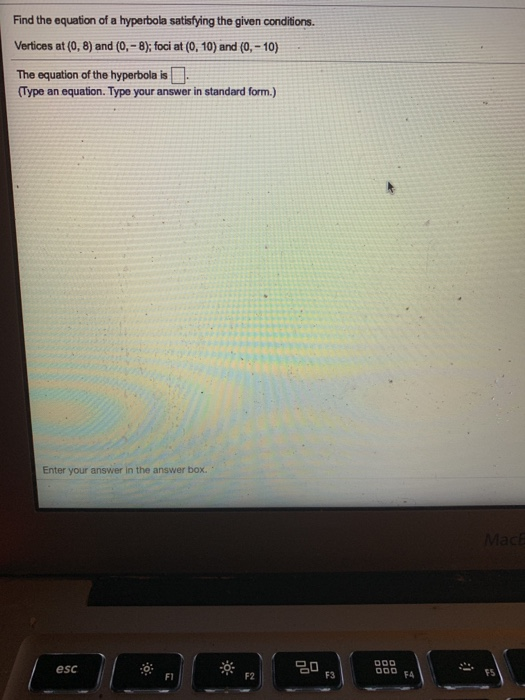Find the equation of a hyperbola satisfying the given conditions. Vertices at (0,8) and (0, - 8); foci at (0, 10) and (0,- 10) The equation of the hyperbola is .. Type an equation. Type your answer in standard form.) Enter your answer in the answer box. MacЕ 000 esc 20 F3 000 FA F1 F2

• ### Question 10 Find the matrix of the quadratic form associated with the quadratic equation 7x2 +1&xy...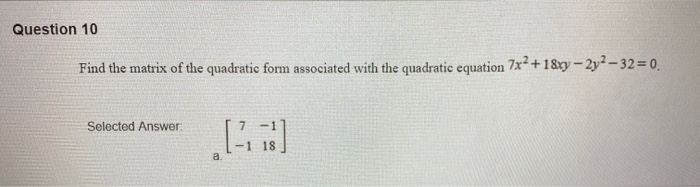Question 10 Find the matrix of the quadratic form associated with the quadratic equation 7x2 +1&xy – 2y2 -32=0. Selected Answer a

• ### Write the standard equation of the ellipse with the given characteristics Vertices: (0,7) (0,-7) foci: (0,2) (0,-2)

Write the standard equation of the ellipse with the given characteristics Vertices: (0,7) (0,-7)foci: (0,2) (0,-2)

• ### Find an equation for the ellipse that satisfies the given conditions. Foci: F(+2, 0), vertices: (3,...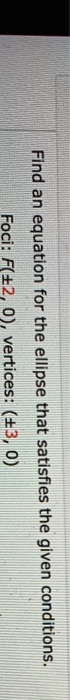Find an equation for the ellipse that satisfies the given conditions. Foci: F(+2, 0), vertices: (3, 0)

• ### Given the equation 3x2 - 12y2 + 6x + 48y - 93 = 0 determine: a)...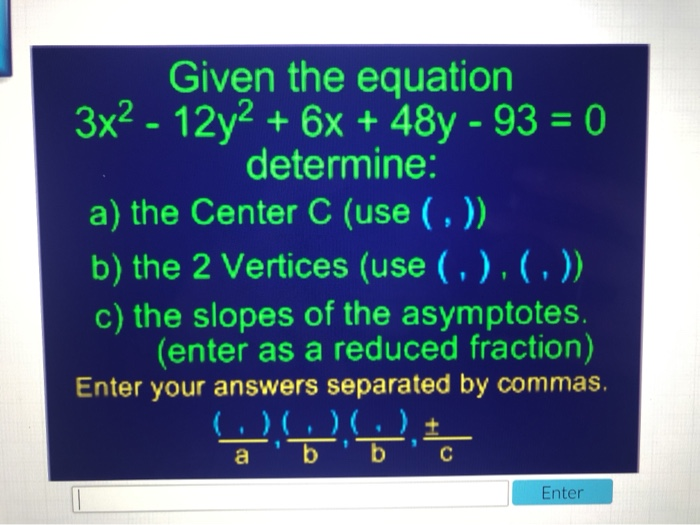Given the equation 3x2 - 12y2 + 6x + 48y - 93 = 0 determine: a) the Center C (use (,)) 'b) the 2 Vertices (use (,).(,)) c) the slopes of the asymptotes. (enter as a reduced fraction) Enter your answers separated by commas, Enter

Free Homework App